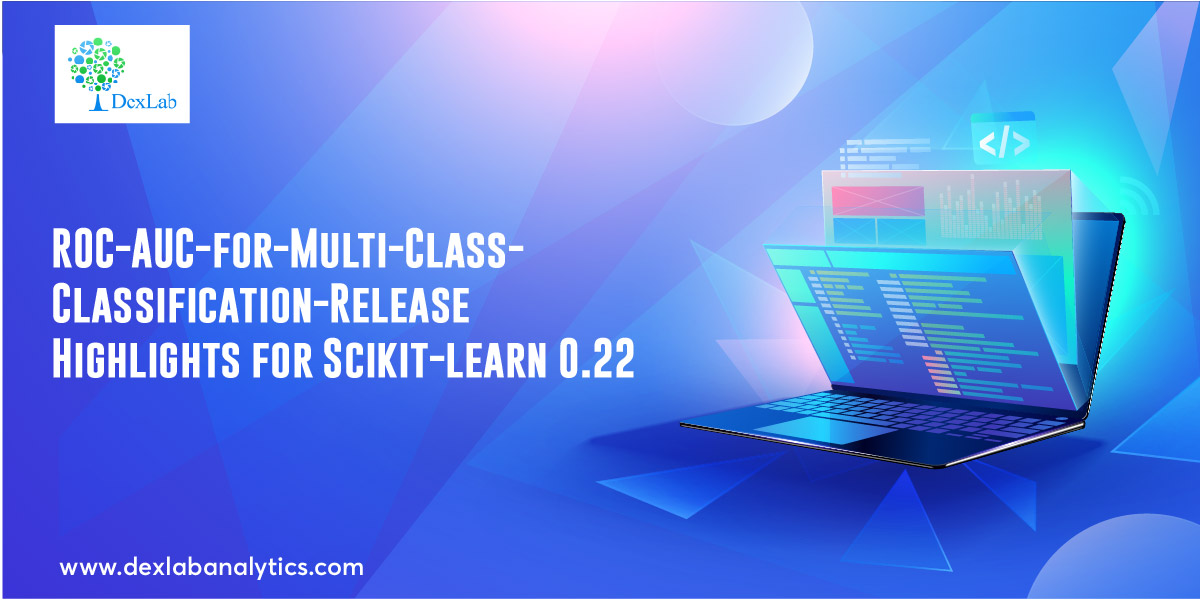# ROC-AUC-for-Multi-Class-Classification-Release Highlights for Scikit-learn 0.22

## Recent PostsToday we are going to learn about the new releases from Scikit-learn version 0.22, a machine learning library in Python. We, through this video tutorial, aim to learn about the much talked about new release wherein ROC-AUC curve supports Multi Class Classification. Prior to this version, Scikit-learn did not have a function to plot the ROC curve.

To access our previous tutorial on the plotting of the ROC curve, click here.

The ROC-AUC score function can also be used in multi-class classification. Two averaging strategies are currently supported: the one-vs-one (OvO) algorithm computes the average of the pairwise ROC AUC scores and the one-vs-rest (OvR) algorithm computes the average of the ROC AUC scores for each class against all other classes.

In both cases, the multiclass ROC AUC scores are computed from probability estimates that a sample belongs to a particular class according to the model. The OvO and OvR algorithms support weighting uniformly (average=’macro’) and weighting by prevalence (average=’weighted’).

To begin with, we import multi classification, SVC and roc_auc_score. Then we specify the number of classes we want in the multi-classification function. Then we apply the SVC function and finally the roc_auc_score one. This function will give us the probable prediction for all the classes and we will then choose the one that has the highest probability. When we run it we get a ROC_AUC score of 0.99.

#### The code sheet is provided in a Github repository here.

For more on this do watch the video attached herewith. This tutorial was brought to you by DexLab Analytics. DexLab Analytics is a premiere Machine Learning institute in Gurgaon.

#### Watch the video here.

.### Call us to know more### Gurgaon+91 931 572 5902